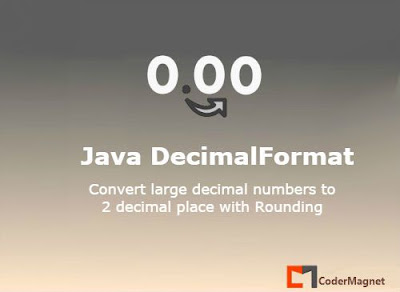•## Wednesday, 26 October 2016

### Java DecimalFormat :Format large numbers to 2 decimal places### Java DecimalFormat - For Large Decimal Point Numbers

The Java DecimalFormat class helps us to format numbers in many ways. I was trying to convert
a big number to a 2 decimal point number.Thought of sharing the same with some explaination.

Trying to achieve this:    1,308,207 ---> 1.31

``````
String number = "1,308,207";
java.text.DecimalFormat df = new java.text.DecimalFormat("#.##");
number = number.replace(",", "");
Float f = Float.parseFloat(number) / 1000000;
String result = df.format(f);
System.out.println(result);   // result = 1.31
``````

#### So how does it work??

Well it works like this. DecimalFromat uses java.math.RoundingMode.HALF_EVEN method which
rounds off the number to nearest even i.e when it's 0.05 it becomes 0.1.

1. The number becomes 1308207 at line 3.
2. At line no. 5 it becomes 1.308207.
3. Now the actual formatting starts:-
4. The right hand side(RHS) value is 07,so nearest round off is 10, only 1 taken & RHS discarded.
5. Now the number becomes 1.308201
6. Rightmost 01,the nearest round off id 00, RHS discarded & number becomes 0.
7. Again number becomes smaller 1.30820, in same way becomes 1.3082.
8. Next step : 1.308
9. Finally 1.31(08 becomes 10 with HALF_EVEN method and 0 discarded).

Do you have suggestions or want to improve the solution? Please do feel free to write back to us